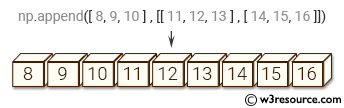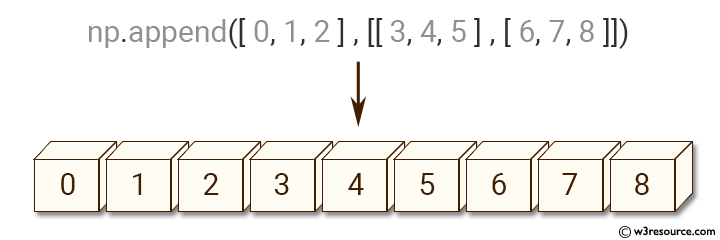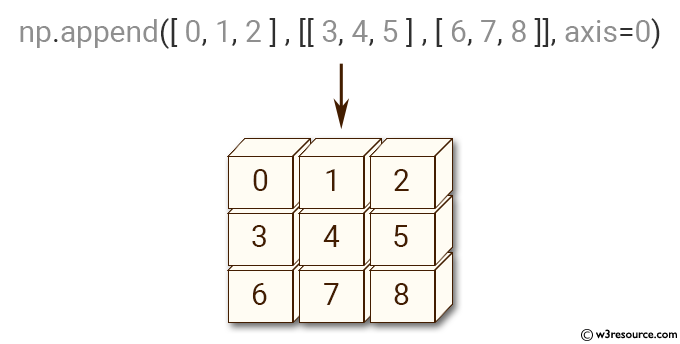# NumPy Array manipulation: append() function

## numpy.append() function

The append() function is used to append values to the end of an given array.

Syntax:

```numpy.append(arr, values, axis=None)
```Version: 1.15.0

Parameter:

Name Description Required /
Optional
arr Values are appended to a copy of this array. Required
values These values are appended to a copy of arr. It must be of the correct shape (the same shape as arr, excluding axis). If axis is not specified, values can be any shape and will be flattened before use. Required
axis The axis along which values are appended. If axis is not given, both arr and values are flattened before use. Optional

Return value:

append : ndarray - A copy of arr with values appended to axis. Note that append does not occur in-place: a new array is allocated and filled. If axis is None, out is a flattened array.

Example-1: numpy.append()

``````>>> import numpy as np
>>> np.append ([0, 1, 2], [[3, 4, 5], [6, 7, 8]])
array([0, 1, 2, 3, 4, 5, 6, 7, 8])
``````

Pictorial Presentation:Example-2: numpy.append()

``````>>> import numpy as np
>>> np.append([[0, 1, 2], [3, 4, 5]],[[6, 7, 8]], axis=0)
array([[0, 1, 2],
[3, 4, 5],
[6, 7, 8]])
``````

Pictorial Presentation:Python - NumPy Code Editor:

Previous: insert()
Next: resize()

﻿